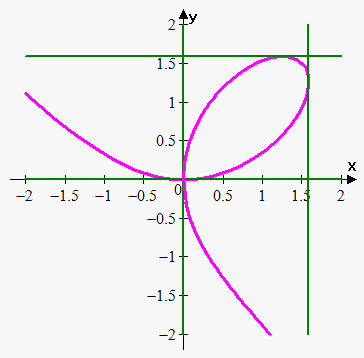# Implicit Differentiation

The techniques we learned allow us to differentiate the functions like ${y}={\sqrt[{{3}}]{{{{x}}^{{2}}+{\sin{{\left({x}\right)}}}}}}$ or ${y}=\frac{{\tan{{\left({x}\right)}}}}{{{{e}}^{{{\sin{{\left({x}\right)}}}}}}}$, or, in general, ${y}={f{{\left({x}\right)}}}$. But what to do if we can't express ${y}$ in terms of ${x}$ or if the expression would be very complex?

Consider ${{x}}^{{2}}+{{y}}^{{2}}={16}$. This is the equation of a circle, and we can solve it for ${y}$: ${y}=\pm\sqrt{{{16}-{{x}}^{{2}}}}$. But here we obtained two functions and we have more work than earlier.

For example, ${{x}}^{{3}}+{{y}}^{{3}}={2}{x}{y}$ can be solved for ${y}$, but the expression would be very complex, and, finally, ${{x}}^{{2}}+{{y}}^{{4}}={\sin{{\left({x}+{y}\right)}}}$ can't be solved explicitly for ${x}$.

What to do in such cases?

Fortunately, we don't need to express ${y}$ in terms of ${x}$. We can use implicit differentiation. This consists in differentiating both sides of the equation with respect to ${x}$ and then solving the resulting equation for ${y}'$. We assume that the given equation determines ${y}$ implicitly as a differentiable function of ${x}$, so that the method of implicit differentiation could be applied.

Example 1. Find the equation of the tangent line to ${{x}}^{{2}}+{{y}}^{{2}}={25}$ at the point $(3,4)$.

To find the slope of the tangent line, we need the derivative of the function. Here, the function is given implicitly; so, we use implicit differentiation.

Differentiate both sides of the equation with respect to ${x}$ and remember that ${y}$ is a function of ${x}$.

$\frac{{d}}{{{d}{x}}}{\left({{x}}^{{2}}+{{y}}^{{2}}\right)}=\frac{{d}}{{{d}{x}}}{\left({25}\right)}$

$\frac{{d}}{{{d}{x}}}{\left({{x}}^{{2}}\right)}+\frac{{d}}{{{d}{x}}}{\left({{y}}^{{2}}\right)}={0}$

${2}{x}+{2}{y}\frac{{{d}{y}}}{{{d}{x}}}={0}$.

Notice how we used the chain rule while differentiating ${{y}}^{{2}}$ : $\frac{{d}}{{{d}{x}}}{\left({{y}}^{{2}}\right)}=\frac{{d}}{{{d}{y}}}{\left({{y}}^{{2}}\right)}\cdot\frac{{{d}{y}}}{{{d}{x}}}={2}{y}\frac{{{d}{y}}}{{{d}{x}}}$.

Solving for $\frac{{{d}{y}}}{{{d}{x}}}$ gives $\frac{{{d}{y}}}{{{d}{x}}}=-\frac{{x}}{{y}}$.

Therefore, at the point $(3,4)$, the slope of the tangent line is $-\frac{{3}}{{4}}$, and the equation of the tangent line is ${y}-{4}=-\frac{{3}}{{4}}{\left({x}-{3}\right)}$, or ${y}=-\frac{{3}}{{4}}{x}+\frac{{25}}{{4}}$.

As can be seen, implicit differentiation can make differentiation easier and can save loads of time.

Example 2. Where is the tangent line horizontal or vertical to ${{x}}^{{3}}+{{y}}^{{3}}={3}{x}{y}$ (folium of Descartes)?

Differentiating both sides of the equation with respect to ${x}$, we obtain $\frac{{d}}{{{d}{x}}}{\left({{x}}^{{3}}+{{y}}^{{3}}\right)}=\frac{{d}}{{{d}{x}}}{\left({3}{x}{y}\right)}$, or $\frac{{d}}{{{d}{x}}}{\left({{x}}^{{3}}\right)}+\frac{{d}}{{{d}{x}}}{\left({{y}}^{{3}}\right)}={3}\frac{{d}}{{{d}{x}}}{\left({x}{y}\right)}$.

To differentiate ${x}{y}$, we need to use the product rule: $\frac{{d}}{{{d}{x}}}{\left({x}{y}\right)}=\frac{{d}}{{{d}{x}}}{\left({x}\right)}{y}+{x}\frac{{d}}{{{d}{x}}}{\left({y}\right)}={y}+{x}\frac{{{d}{y}}}{{{d}{x}}}$.

To differentiate ${{y}}^{{3}}$, we need to use the chain rule: $\frac{{d}}{{{d}{x}}}{{y}}^{{3}}=\frac{{d}}{{{d}{y}}}{\left({{y}}^{{3}}\right)}\cdot\frac{{{d}{y}}}{{{d}{x}}}={3}{{y}}^{{2}}\frac{{{d}{y}}}{{{d}{x}}}$.

So, the equation can be rewritten as

${3}{{x}}^{{2}}+{3}{{y}}^{{2}}\frac{{{d}{y}}}{{{d}{x}}}={3}{\left({y}+{x}\frac{{{d}{y}}}{{{d}{x}}}\right)}$.

Solving for $\frac{{{d}{y}}}{{{d}{x}}}$ gives $\frac{{{d}{y}}}{{{d}{x}}}=\frac{{{y}-{{x}}^{{2}}}}{{{{y}}^{{2}}-{x}}}$.The tangent line is horizontal when $\frac{{{d}{y}}}{{{d}{x}}}={0}$, or ${y}-{{x}}^{{2}}={0}$, which gives ${y}={{x}}^{{2}}$.

If we now substitute ${y}$ in the initial equation with the obtained expression, we have that ${{x}}^{{3}}+{{\left({{x}}^{{2}}\right)}}^{{3}}={3}{x}\cdot{{x}}^{{2}}$, or ${{x}}^{{6}}-{2}{{x}}^{{3}}={0}$.

Thus, the tangent line is horizontal when either ${x}={0}$ or ${x}={{2}}^{{\frac{{1}}{{3}}}}$.

So, the tangent line is horizontal at the points $(0,0)$ and ${\left({{2}}^{{\frac{{1}}{{3}}}},{{\left({{2}}^{{\frac{{1}}{{3}}}}\right)}}^{{2}}\right)}={\left({{2}}^{{\frac{{1}}{{3}}}},{{2}}^{{\frac{{2}}{{3}}}}\right)}\approx{\left({1.26},{1.59}\right)}$.

The tangent line is vertical when the denominator in the expression for $\frac{{{d}{y}}}{{{d}{x}}}$ is $0$, or ${{y}}^{{2}}={x}.$ Another method is to observe that the equation of the curve is unchanged when ${x}$ and ${y}$ are interchanged, so the curve is symmetric about the line ${y}={x}$. This means that the horizontal tangents at $(0, 0)$ and ${\left({{2}}^{{\frac{{1}}{{3}}}},{{2}}^{{\frac{{2}}{{3}}}}\right)}$ correspond to the vertical tangents at $(0, 0)$ and ${\left({{2}}^{{\frac{{2}}{{3}}}},{{2}}^{{\frac{{1}}{{3}}}}\right)}$.

Example 3. Find ${y}'=\frac{{{d}{y}}}{{{d}{x}}}$, where ${\sin{{\left({x}+{y}\right)}}}={{e}}^{{{{x}}^{{2}}{{y}}^{{2}}}}$.

We use the chain rule and the product rule.

$\frac{{d}}{{{d}{x}}}{\left({\sin{{\left({x}+{y}\right)}}}\right)}=\frac{{d}}{{{d}{x}}}{\left({{e}}^{{{{x}}^{{2}}{{y}}^{{2}}}}\right)}$

${\cos{{\left({x}+{y}\right)}}}\cdot\frac{{d}}{{{d}{x}}}{\left({x}+{y}\right)}={{e}}^{{{{x}}^{{2}}{{y}}^{{2}}}}\cdot\frac{{d}}{{{d}{x}}}{\left({{x}}^{{2}}{{y}}^{{2}}\right)}$

${\cos{{\left({x}+{y}\right)}}}{\left({1}+{y}'\right)}={{e}}^{{{{x}}^{{2}}{{y}}^{{2}}}}{\left(\frac{{d}}{{{d}{x}}}{\left({{x}}^{{2}}\right)}{{y}}^{{2}}+{{x}}^{{2}}\frac{{d}}{{{d}{x}}}{\left({{y}}^{{2}}\right)}\right)}$

${\cos{{\left({x}+{y}\right)}}}{\left({1}+{y}'\right)}={{e}}^{{{{x}}^{{2}}{{y}}^{{2}}}}{\left({2}{x}{{y}}^{{2}}+{2}{y}{{x}}^{{2}}{y}'\right)}$

${\cos{{\left({x}+{y}\right)}}}+{\cos{{\left({x}+{y}\right)}}}{y}'={2}{x}{{y}}^{{2}}{{e}}^{{{{x}}^{{2}}{{y}}^{{2}}}}+{2}{y}{{x}}^{{2}}{{e}}^{{{{x}}^{{2}}{{y}}^{{2}}}}{y}'$.

Solving for ${y}'$ gives ${y}'=\frac{{{\cos{{\left({x}+{y}\right)}}}-{2}{x}{{y}}^{{2}}{{e}}^{{{{x}}^{{2}}{{y}}^{{2}}}}}}{{{2}{y}{{x}}^{{2}}{{e}}^{{{{x}}^{{2}}{{y}}^{{2}}}}-{\cos{{\left({x}+{y}\right)}}}}}$.

Since, from the equation of a curve, ${\sin{{\left({x}+{y}\right)}}}={{e}}^{{{{x}}^{{2}}{{y}}^{{2}}}}$, we can write equivalently ${y}'=\frac{{{\cos{{\left({x}+{y}\right)}}}-{2}{x}{{y}}^{{2}}{\sin{{\left({x}+{y}\right)}}}}}{{{2}{y}{{x}}^{{2}}{\sin{{\left({x}+{y}\right)}}}-{\cos{{\left({x}+{y}\right)}}}}}$.